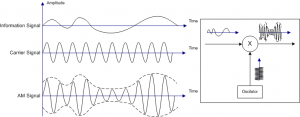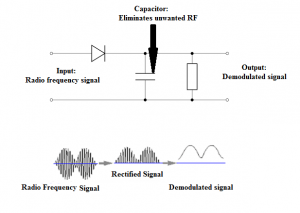# Amplitude Modulation And Demodulation

Amplitude modulation is an electronic communication system technique wherein the baseband signal is superimposed with the amplitude of the carrier wave i.e., the amplitude of the carrier wave is varying proportionally to the base waveform being transmitted. Amplitude modulation has been in use since the earliest days of radio technology. Here we will discuss how to detect amplitude modulated wave.

## What is Amplitude Modulation?

AM is the simplest way of modulating a signal. Production of amplitude modulated signals is easy. Also, the requirements are feasible. When an amplitude modulated signal is created, the amplitude of the created signal represents the original baseband signal to be transmitted. This amplitude forms an envelope over the underlying high-frequency carrier wave. Here, the overall envelope of the carrier is modulated to carry the audio signal. However, AM waves are interrupted by electrical and other disturbances which make them weak and noisy.## Amplitude Modulation Formula

 $s(t)=[A_{c}+A_{m}cos(2\pi f_{m}t)]cos(2\pi f_{c}t)$

Where,

• Am is the amplitude of the modulating signal
• Ac is the amplitude of the carrier signal
• fm is the frequency of the modulating signal
• fc is the frequency of the carrier signal

## Detection of Amplitude Modulated Wave (Demodulation)

Demodulation or detection is a process where the signal that is a mixture of the amplitude of the baseband signal and the frequency of the carrier signal, is deconstructed to yield the original signal that is to be transmitted. Simply, it is the recovery of the modulating signal from the modulated wave.Amplitude modulation and demodulation are equally simple to perform. The amplitude modulated signal needs just a simple diode detector circuit to demodulate. The diode rectifies the incoming signal, allowing only one-half of the signal waveform to pass through. The capacitor then is used to remove the radio frequency parts of the signal, leaving just the original waveform. As you see, the equipment for demodulation is very cheap, and this enables the cost of the receivers to be kept low.

Thus, amplitude modulated wave can be demodulated in two steps:

• Rectification of modulated wave.
• Elimination of the RF component of the modulated wave.

Watch video lessons on amplitude modulation by downloading BYJU’S – The Learning App.

#### Practise This Question

What is the modulation index of an over modulated wave